You are here: Start » AVL.NET » Image Point Transforms

# Image Point Transforms

Icon Name DescriptionAbsoluteValueImage Transforms pixel values to their absolute values pixel by pixel.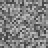AddNoiseToImage Adds random noise to the image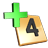AddToImage Adds a scalar value to each pixel.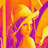ColorizeImage Shows a monochromatic image in false colors.CorrectGamma Image enhancement for human perception. For computer vision consider LogarithmImage.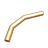CropPixels Sets pixels below the low value to the low value and above the high value to the high value.DivideImage Divides each pixel by a scalar value.InvertImage Applies numeric inversion (1/x) of the pixel values.LogarithmImage Transforms an image in such a way that a quotient on the input image becomes a difference on the output image. This can be useful for dealing with variable illumination.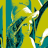LUTTransformImage Changes pixel values for data stored in array.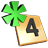MultiplyImage Multiplies each pixel by a scalar value.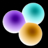NegateImage Reverts the pixel value range (unsigned) or applies numeric negation (signed).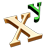PowerImage Exponentiates each pixel to the given power.ReplacePixels Substitutes pixels having the specified value with a new value.ResaturateImage Sets pixels below the low value to minimum, above the high value to maximum, and interpolates the rest.RescalePixels Applies linear transformation to pixel values.SquareImage Raises pixel values to the second power pixel by pixel.SquareRootImage Transforms pixel values to their square roots pixel by pixel.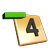SubtractFromImage Subtracts a scalar value from each pixel.